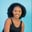Related Tags

python
python3
numpy

# How to use the np.floor_divide() function for 2D array in PythonMaria Elijah

## Overview

The python library NumPy has a method called floor_divide() which can be used to divide two input arrays.

### The numpy.floor_divide() method

The numpy.floor_divide() method divides the first array’s elements by the elements of the second array. This is done element-wise (element by element).

Note: A two-dimensional (2D) array can be created using a list of lists in Python.

### Syntax

numpy.floor_divide(x1, x2, dtype=None, out=None)


### Parameters

• x1: This represents the input data.
• x2: This represents the input data.
• dtype: This is an optional parameter. It represents the return type of the array.
• out: This is an optional parameter. It represents the alternative output array in which the result is to be placed.

Note: If x1 and x2 have distinct shapes, they must be able to be broadcasted to a common shape for output representation.

### Return value

The numpy.floor_divide() method returns a floor array of (x1/x2).

Note: In Python, the operator // is equivalent to the function floor_divide().

### Example

The following code shows how to use the numpy.floor_divide() method for two-dimensional(2D) arrays.

# import numpy
import numpy as np
# create a two 2D arrays
x1 = np.array([[2,6,5],[3,4,8]])
x2 = np.array([[1,7,2],[10,9,4]])

# divide the 2D arrays
# and store the result in result
result = np.floor_divide(x1, x2)

print(result)
Using the np.floor_divide() function

### Explanation

• Line 2: We import the numpy library.
• Lines 4–5: We create two 2D arrays called x1 and x2.
• Line 9: We use the np.floor_divider() which divides the array x1 by x2. The result is stored in a new variable called result.
• Line 11: We display the result.

RELATED TAGS

python
python3
numpy

CONTRIBUTORMaria Elijah
RELATED COURSES

View all Courses

Keep Exploring

Learn in-demand tech skills in half the time